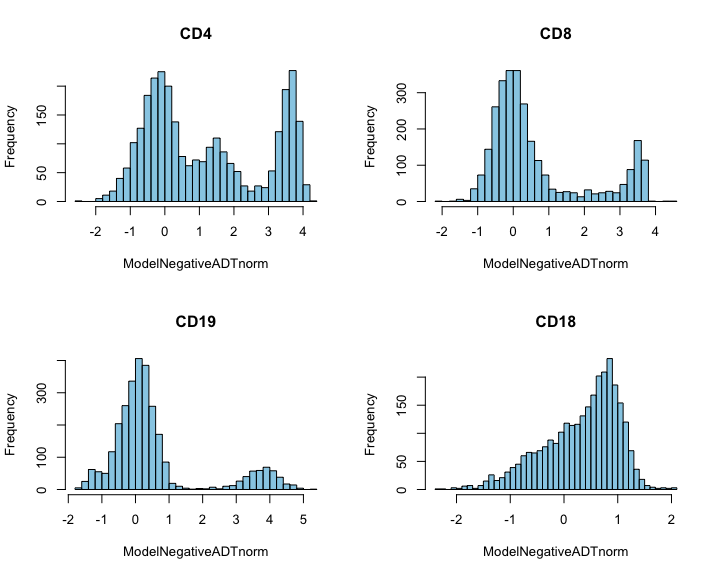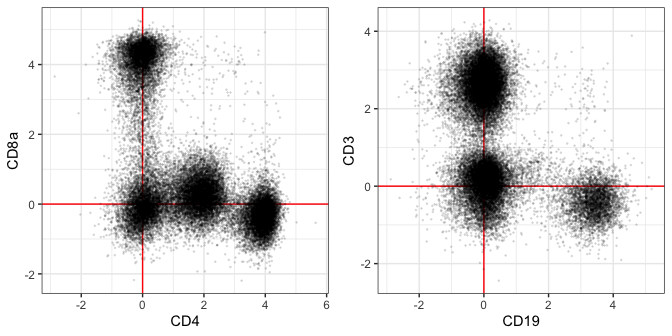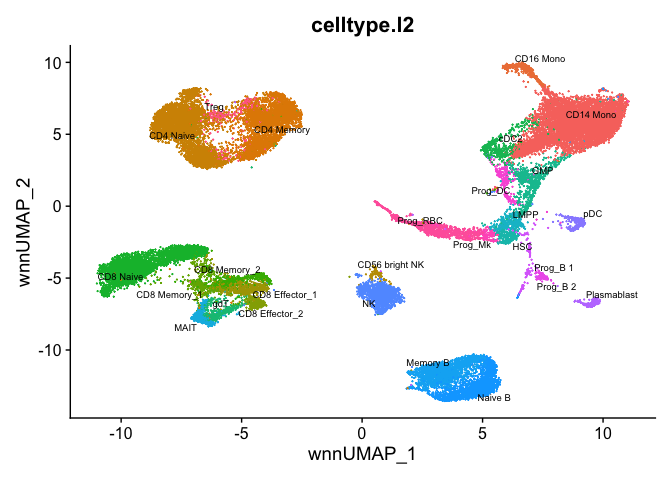This method has performed well on 4 datasets but it has not been as extensively tested as the default DSBNormalizeProtein method.

Sometimes empty droplets are not available. In Supplementary Figure 1, we show that the fitted background population mean of each protein across all cells was concordant with the mean of ambient ADTs in both empty droplets and unstained control cells.

This experiment suggests that this fitted background mean captures an estimate of ambient noise. By log + 1 transforming ADTs across cells, fitting the background population mean with a Gaussian Mixture and subtracting this value from cells, we should partly remove the ambient component and 0-center the background population for each ADT. We can then implement step II exactly as in the dsb function DSBNormalizeProtein. We provide this method with the function ModelNegativeADTnorm.

library(dsb)
# pecify isotype controls to use in step II
isotypes = c("MouseIgG1kappaisotype_PROT", "MouseIgG2akappaisotype_PROT",
"Mouse IgG2bkIsotype_PROT", "RatIgG2bkIsotype_PROT")

# run ModelNegativeADTnorm to model ambient noise and implement step II
denoise.counts = TRUE,
use.isotype.control = TRUE,
isotype.control.name.vec = isotypes
)
#>  "fitting models to each cell for dsb technical component and removing cell to cell technical noise"

We retain trimodal distributions of CD4 with the background population centered at 0. All populations are nicely recovered with concordant distribution of values as the default dsb method.

par(mfrow = c(2,2)); r = '#009ACD80'
hist(norm.adt["CD4_PROT", ], breaks = 45, col = r, main = 'CD4', xlab = lab)
hist(norm.adt["CD8_PROT", ], breaks = 45, col = r, main = 'CD8', xlab = lab)
hist(norm.adt["CD19_PROT", ], breaks = 45, col = r, main = 'CD19', xlab = lab)
hist(norm.adt["CD18_PROT", ], breaks = 45, col = r, main = 'CD18', xlab = lab)Note that two proteins in this 87 protein panel are expected to be expressed by every cell–HLA-ABC and CD18. cells with the lowest expression of ubiquitously expressed proteins can fall around 0 with this method. Relative CD18 values above are the same as the default dsb, however, the lowest population cells with this method are around 0.

Clustering performance and modeling e.g. with protein or joint WNN clustering is highly concordant using the default dsb and this modeled background method. Below we demonstrate WNN using this method on bone marrow CITE-seq data from the Satija lab.

# these data were installed with the SeuratData package
# devtools::install_github('satijalab/seurat-data')
# library(SeuratData)
# InstallData(ds = 'bmcite')

# load bone marrow CITE-seq data
data('bmcite')
bm = bmcite; rm(bmcite)

# Extract raw bone marrow ADT data

# unfortunately this data does not have isotype controls
denoise.counts = TRUE,
use.isotype.control = FALSE)

Above we removed technical cell to cell variations with each cells fitted background only–adding isotype controls will further improve the precision in the technical component estimation. Note, if this dataset included isotype controls we would have used the following options:


# specify isotype controls
isotype.controls = c('isotype1', 'isotype 2')
denoise.counts = TRUE,
use.isotype.control = TRUE,
isotype.control.name.vec = isotype.controls
)

Examine protein expression distributions:

library(ggplot2); theme_set(theme_bw())
plist = list(geom_vline(xintercept = 0, color = 'red'),
geom_hline(yintercept = 0, color = 'red'),
geom_point(size = 0.2, alpha = 0.1))
d = as.data.frame(t(dsb.norm))

# plot distributions
p1 = ggplot(d, aes(x = CD4, y = CD8a)) + plist
p2 = ggplot(d, aes(x = CD19, y = CD3)) + plist
cowplot::plot_grid(p1,p2)Now we can cluster with WNN using the dsb normalized values as in the other end to end analysis vignette. We first add the dsb normalized values to the object directly and we do not normalize with CLR, instead proceeding directly with the Seurat WNN clustering pipeline.

bm = SetAssayData(bmcite, slot = 'data',
new.data = dsb.norm)

# process RNA for WNN
DefaultAssay(bm) <- 'RNA'
bm <- NormalizeData(bm) %>%
FindVariableFeatures() %>%
ScaleData() %>%
RunPCA()

# process ADT for WNN # see the main dsb vignette for an alternate version
bm = bm %>% ScaleData() %>% RunPCA(reduction.name = 'apca')

# run WNN
bm <- FindMultiModalNeighbors(
bm, reduction.list = list("pca", "apca"),
dims.list = list(1:30, 1:18), modality.weight.name = "RNA.weight"
)

bm <- FindClusters(bm, graph.name = "wsnn",
algorithm = 3, resolution = 2,
verbose = FALSE)
bm <- RunUMAP(bm, nn.name = "weighted.nn",
reduction.name = "wnn.umap",
reduction.key = "wnnUMAP_")

p2 <- DimPlot(bm, reduction = 'wnn.umap',
group.by = 'celltype.l2',
label = TRUE, repel = TRUE,
label.size = 2.5) + NoLegend()
p2